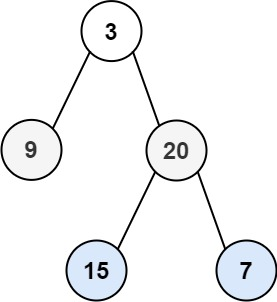| English | 简体中文 |

# 103. Binary Tree Zigzag Level Order Traversal

## Description

Given the root of a binary tree, return the zigzag level order traversal of its nodes' values. (i.e., from left to right, then right to left for the next level and alternate between).

Example 1:Input: root = [3,9,20,null,null,15,7]
Output: [,[20,9],[15,7]]


Example 2:

Input: root = 
Output: []


Example 3:

Input: root = []
Output: []


Constraints:

• The number of nodes in the tree is in the range [0, 2000].
• -100 <= Node.val <= 100